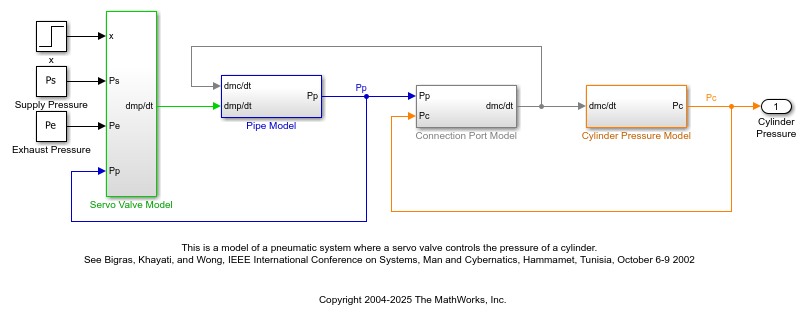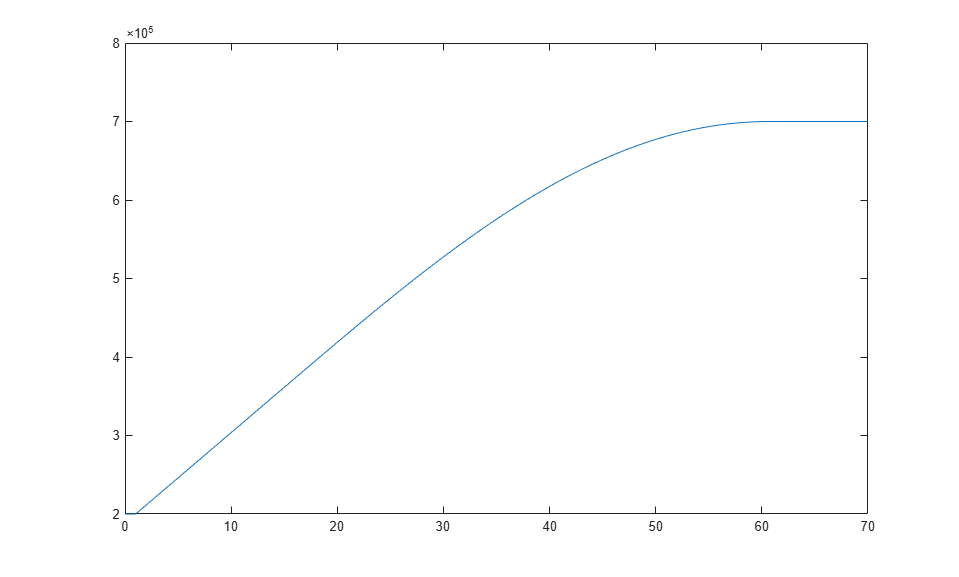# Linearize Pneumatic System at Simulation Snapshots

This example shows how to linearize a Simulink® model at time-based operating point snapshots. The example uses a model of the dynamics of filling a cylinder with compressed air.

### Pneumatic System Model

```mdl = 'scdpneumaticlin'; open_system(mdl) ```Simulate the model.

```[t,x,y] = sim(mdl); ```

In this example, the supply pressure is closed and the system has an initial pressure of 0.2 MPa. The supply pressure is at 0.7 MPa. In the simulation, the servo valve is opened to 0.5e-4 m. During the simulation, the pressure increases from the initial pressure of 0.2 MPa and eventually settles at the supply pressure.

```plot(t,y) ```### Take Simulation Snapshots

Compute operating points at multiple simulation times from 0 to 60 seconds in 10-second intervals. The `findop` function simulates the model, takes a snapshot of the model conditions at each simulation time, and computes an operating point for each snapshot.

```op = findop(mdl,[0 10 20 30 40 50 60]); ```

View the operating point for the second snapshot time.

```op(2) ```
```ans = Operating point for the Model scdpneumaticlin. (Time-Varying Components Evaluated at time t=10.7245) States: ---------- <strong>x</strong> <strong>__________</strong> (1.) scdpneumaticlin/Cylinder Pressure Model/dPc//dt 3.1205e+05 (2.) scdpneumaticlin/Pipe Model/dPp//dt 3.1251e+05 Inputs: None ---------- ```

### Linearize Model

To linearize the model, first specify the linearization input and output points. For this example, linearize the model from servo valve opening `x` to the output pressure.

```io(1) = linio('scdpneumaticlin/x',1,'input'); io(2) = linio('scdpneumaticlin/Cylinder Pressure Model',1,'output'); ```

Linearize the model for all of the computed snapshots. `sys` is an array of state-space models.

```sys = linearize(mdl,op,io); ```

To see the variability in the linearizations, plot the frequency responses of the resulting linear systems.

```bode(sys) ```Close the model.

```bdclose(mdl) ```